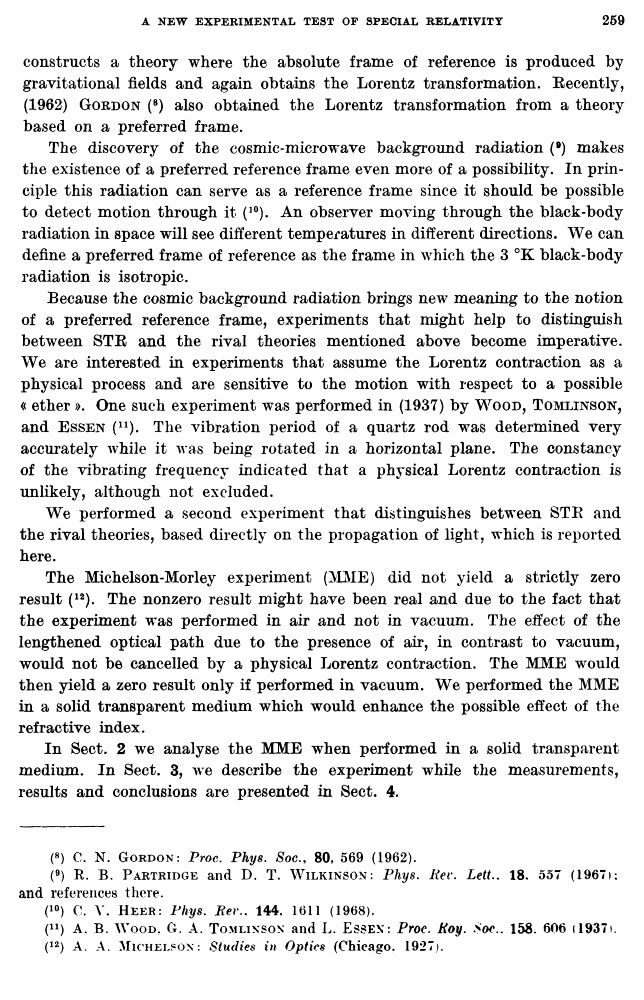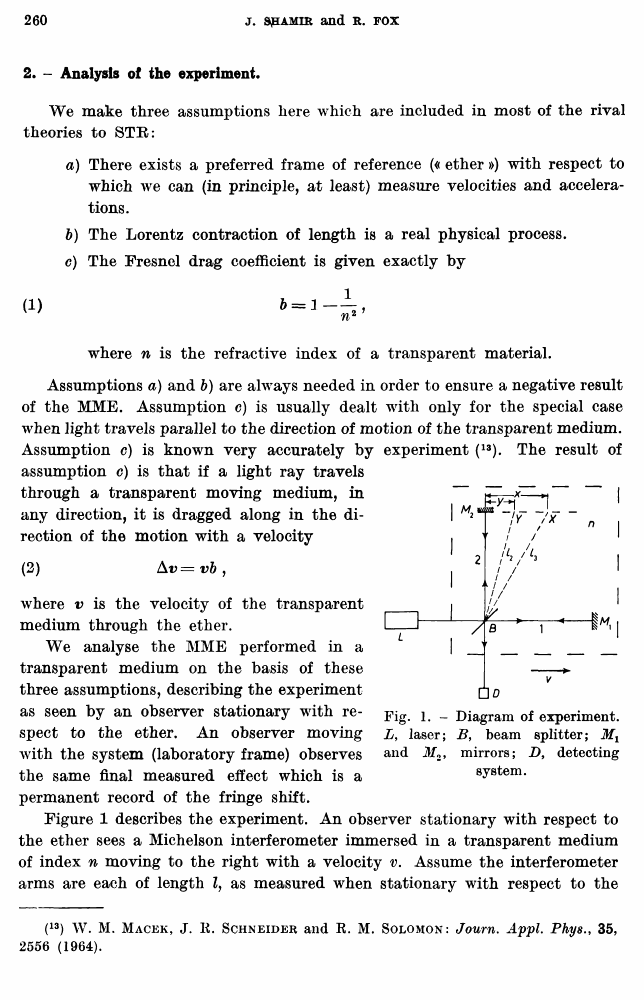Shamir, J.; Fox, R. A new experimental test of special relativity // Nuovo Cimento B Series 10, vol. 62, issue 2, 11 Aug. 1969

В начало   Другие форматы   <<<     Страница 259   >>>258  259 260  261  262  263  264

constructs a theory where the absolute frame of reference is produced by gravitational fields and again obtains the Lorentz transformation. Eecently, (1962) Gordon (8) also obtained the Lorentz transformation from a theory based on a preferred frame.

The discovery of the cosmic-micro wave background radiation (9) makes the existence of a preferred reference frame even more of a possibility. In principle this radiation can serve as a reference frame since it should be possible to detect motion through it (10). An observer moving through the black-body radiation in space will see different temperatures in different directions. We can define a preferred frame of reference as the frame in which the 3 °K black-body radiation is isotropic.

Because the cosmic background radiation brings new meaning to the notion of a preferred reference frame, experiments that might help to distinguish between STB and the rival theories mentioned above become imperative. We are interested in experiments that assume the Lorentz contraction as a physical process and are sensitive to the motion with respect to a possible « ether ». One such experiment was performed in (1937) by Wood, Tomlinson, and Essen (11). The vibration period of a quartz rod was determined very accurately while it was being rotated in a horizontal plane. The constancy of the vibrating frequency indicated that a physical Lorentz contraction is unlikely, although not excluded.

We performed a second experiment that distinguishes between STB and the rival theories, based directly on the propagation of light, which is reported here.

The Michelson-Morley experiment (MME) did not yield a strictly zero result (12). The nonzero result might have been real and due to the fact that the experiment was performed in air and not in vacuum. The effect of the lengthened optical path due to the presence of air, in contrast to vacuum, would not be cancelled by a physical Lorentz contraction. The MME would then yield a zero result only if performed in vacuum. We performed the MME in a solid transparent medium which would enhance the possible effect of the refractive index.

In Sect. 2 we analyse the MME when performed in a solid transparent medium. In Sect. 3, we describe the experiment while the measurements, results and conclusions are presented in Sect. 4.

(8) C. N. Gordon: Proc. Phys. Soc80, 569 (1962).

(9) R. B. Partridge and D. T. Wilkinson: Phys. Rev. Lett.. 18. 557 (1967): and references there.

(10) C. V. Heer: Phys. Her.. 144, 1611 (1968).

(n) A. B. Wood, G. A. Tomlinson and L. Essex: Proc. Roy. Soc.. 158. 606 (1937i.

(12) A. A. Michelson: Studies in Optics (Chicago. 1927).

2. - Analysis of the experiment.

We make three assumptions here which are included in most of the rival theories to STR:

a) There exists a preferred frame of reference (« ether ») with respect to which we can (in principle, at least) measure velocities and accelerations.

b) The Lorentz contraction of length is a real physical process.

c) The Fresnel drag coefficient is given exactly by

where n is the refractive index of a transparent material.

Assumptions a) and b) are always needed in order to ensure a negative result of the MME. Assumption c) is usually dealt with only for the special case when light travels parallel to the direction of motion of the transparent medium. Assumption c) is known very accurately by experiment (13). The result of assumption c) is that if a light ray travels through a transparent moving medium, in any direction, it is dragged along in the direction of the motion with a velocity

(2) Av = vb ,

where v is the velocity of the transparent medium through the ether.

We analyse the MME performed in a transparent medium on the basis of these three assumptions, describing the experiment as seen by an observer stationary with respect to the ether. An observer moving with the system (laboratory frame) observes the same final measured effect which is a permanent record of the fringe shift.

Figure 1 describes the experiment. An observer stationary with respect to the ether sees a Michelson interferometer immersed in a transparent medium of index n moving to the right with a velocity v. Assume the interferometer arms are each of length Z, as measured when stationary with respect to the

(13) W. M. Macek, J. E. Schneider and E. M. Solomon: Journ. Appl. Phys., 35, 2556 (1964).

Fig. 1. - Diagram of experiment. L, laser; B, beam splitter; Mx and Jf2, mirrors; Z>, detecting system.